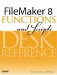# Tan()

Category: Trigonometric

Parameters:

numberAny number representing the size of an angle measured in radians.

Data type returned: Number

Description:

Returns the tangent of the specified angle.

The tangent of an angle can also be obtained by dividing the sine of the angle by its cosine. In any right triangle, the tangent of the two non-right angles can be obtained by dividing the length of the side opposite the angle by the length of the adjacent side.

Examples:

Function

Results

Tan (0)

Returns 0.

Tan (Pi / 6)

Returns .5773502691896257.

Returns 1.FileMaker 8 Functions and Scripts Desk Reference
ISBN: 0789735113
EAN: 2147483647
Year: 2004
Pages: 352